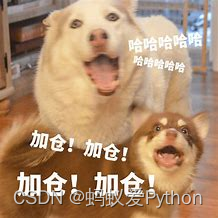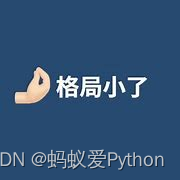# Python 最有用的25个代码段

## 前言

Python是一种通用的高级编程语言。用它可以做许多事，比如开发桌面 GUI 应用程序、网站和 Web 应用程序等。

``````1.与主要平台和操作系统兼容；

2.有许多开源框架和工具；

3.代码具备可读性和可维护性；

4.健壮的标准库；

5.标准测试驱动开发
````````````1.在两个变量之间交换值
``````

Python 中，它就简单多了，如下所示。

``````a = 5
b = 10
a,b = b,
aprint(a) # 10
print(b) # 5
``````

## 2.检查给定的数字是否为偶数

``````python学习交流Q群：906715085###
def is_even(num):
return num % 2 == 0
is_even(10) # True
``````

## 3.将多行字符串拆分为行列表

``````def split_lines(s):
return s.split('\n')
split_lines('50\n python\n snippets') # ['50', ' python', ' snippets']
``````

## 4.查找对象使用的内存

``````import sys
print(sys.getsizeof(5)) # 28
print(sys.getsizeof("Python")) # 55
``````

## 5.反转字符串

Python 字符串库不像其他 Python 容器（如 list) 那样支持内置的 reverse()。反转字符串有很多方法，其中最简单的方法是使用切片运算符（slicing operator）。

``````language = "python"
reversed_language = language[::-1]
print(reversed_language) # nohtyp
``````

## 6.打印字符串 n 次

``````def repeat(string, n):
return (string * n)
repeat('python', 3)
# pythonpythonpython
``````## 7.检查字符串是否为回文

``````def palindrome(string):
return string == string[::-1]
palindrome('python') # False
``````

## 8.将字符串列表合并为单个字符串

``````strings = ['50', 'python', 'snippets']
print(','.join(strings)) # 50,python,snippets
``````

## 9.查找列表的第一个元素

``````def head(list):
return list
print(head([1, 2, 3, 4, 5])) # 1
``````

## 10.查找存在于两个列表中任一列表存在的元素

``````def union(a,b):
return list(set(a + b))
union([1, 2, 3, 4, 5], [6, 2, 8, 1, 4]) # [1,2,3,4,5,6,8]
``````

## 11.查找给定列表中的所有唯一元素

``````def unique_elements(numbers):
return list(set(numbers))
unique_elements([1, 2, 3, 2, 4]) # [1, 2, 3, 4]
``````

## 12.求一组数字的平均值

``````def average(*args):
return sum(args, 0.0) / len(args)
average(5, 8, 2) # 5.0
``````

## 13.检查列表是否包含所有唯一值

``````def unique(list):
if len(list)==len(set(list)):
print("All elements are unique")
else:
print("List has duplicates")
unique([1,2,3,4,5]) # All elements are unique
``````## 14.跟踪列表中元素的频率

Python 计数器跟踪容器中每个元素的频率。Counter() 返回一个以元素为键、以其出现频率为值的字典。

``````from collections import Counte
rlist = [1, 2, 3, 2, 4, 3, 2, 3]
count = Counter(list)
print(count) # {2: 3, 3: 3, 1: 1, 4: 1}
``````

## 15.查找列表中最常用的元素

``````def most_frequent(list):
return max(set(list), key = list.count)

numbers = [1, 2, 3, 2, 4, 3, 1, 3]
most_frequent(numbers) # 3
``````

## 16.将角度转换为弧度

``````import math
return (deg * math.pi) / 180.0

``````

## 17.计算执行一段代码所需的时间

``````import time
start_time = time.time()
a,b = 5,10
c = a+b
end_time = time.time()time_taken = (end_time- start_time)*(10**6)
print("Time taken in micro_seconds:", time_taken) # Time taken in micro_seconds: 39.577484130859375
``````

## 18.查找数字列表的最大公约数

``````from functools
import reduceimport mathdef gcd(numbers):
return reduce(math.gcd, numbers)gcd([24,108,90]) # 6
``````## 19.查找字符串中的唯一字符

``````string = "abcbcabdb"
unique = set(string)new_string = ''.
join(unique)print(new_string) # abcd
``````

## 20.使用 lambda 函数

Lambda 是一个匿名函数，它只能保存一个表达式。

``````x = lambda a, b, c : a + b + c
print(x(5, 10, 20)) # 35
``````

## 标题21.使用映射函数

``````def multiply(n):
return n * n
list = (1, 2, 3)
result = map(multiply, list)
print(list(result)) # {1, 4, 9}
``````

## 22.使用筛选函数

``````arr = [1, 2, 3, 4, 5]
arr = list(filter(lambda x : x%2 == 0, arr))
print (arr) # [2, 4]
``````

## 23.使用列表解析

``````numbers = [1, 2, 3]
squares = [number**2 for number in numbers]
print(squares) # [1, 4, 9]
``````

## 24.使用切片运算符

``````def rotate(arr, d):
return arr[d:] + arr[:d]
if __name__ == '__main__':
arr = [1, 2, 3, 4, 5]
arr = rotate(arr, 2)
print (arr) # [3, 4, 5, 1, 2]
``````

## 25.使用函数链式调用

``````def add(a, b):
return a + bdef subtract(a, b):
return a - ba, b = 5, 10
print((subtract if a > b else add)(a, b)) # 15
``````